BROWSE BY

#### Author

Results 1 - 7 of 7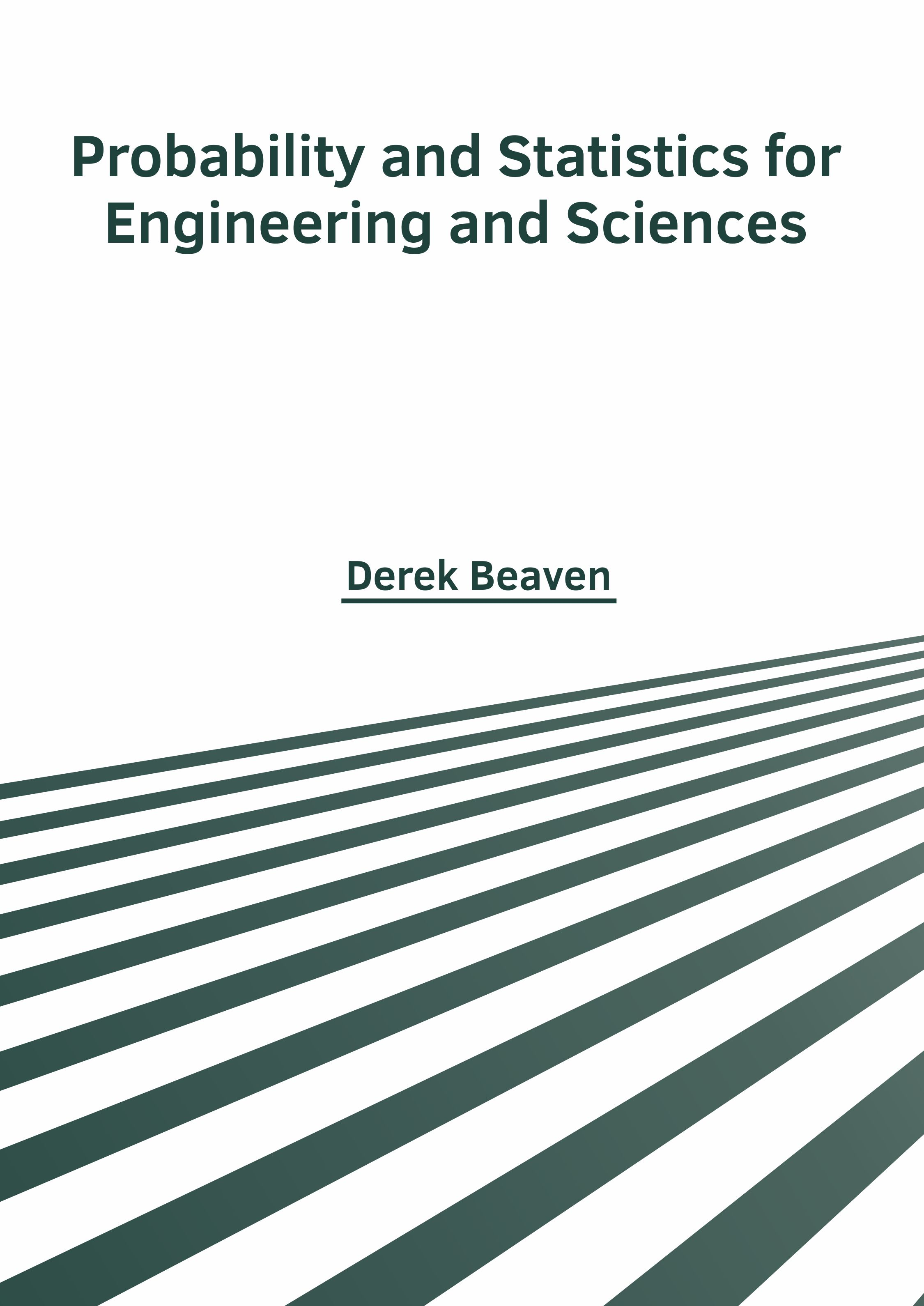Probability and Statistics for Engineering and Sciences Author : Derek Beaven Subject : Probability and Statistics Probability and Statistics are two closely related sub-disciplines of mathematical. Statistics is a mathematical branch that deals with data collection, organization, interpretation, presentation and Read More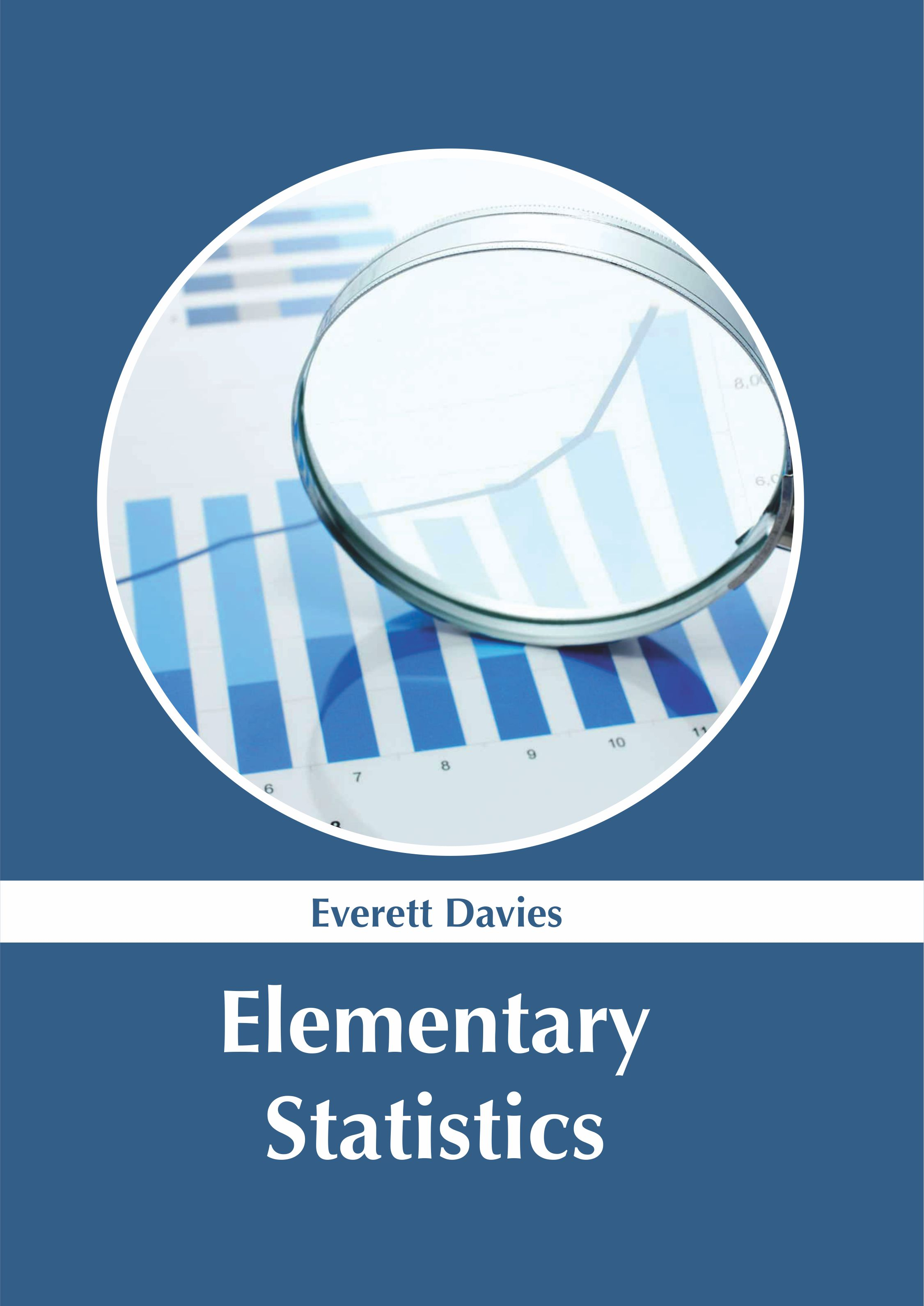Elementary Statistics Author : Everett Davies Subject : Probability and Statistics ISBN :9781632387059 Statistics is a branch of mathematics, which deals with the collection, analysis, presentation and organization of data. It can involve the design of surveys and experiments for the collection of data. Read MoreProbability and Statistics: Theories and Applied Principles Author : Derek Beaven Subject : Probability and Statistics ISBN :9781632385802 The subjects of statistics and probability are interrelated and are generally studied together. They can be applied in a number of fields such as finance, machine learning, game theory, etc. The book presents Read More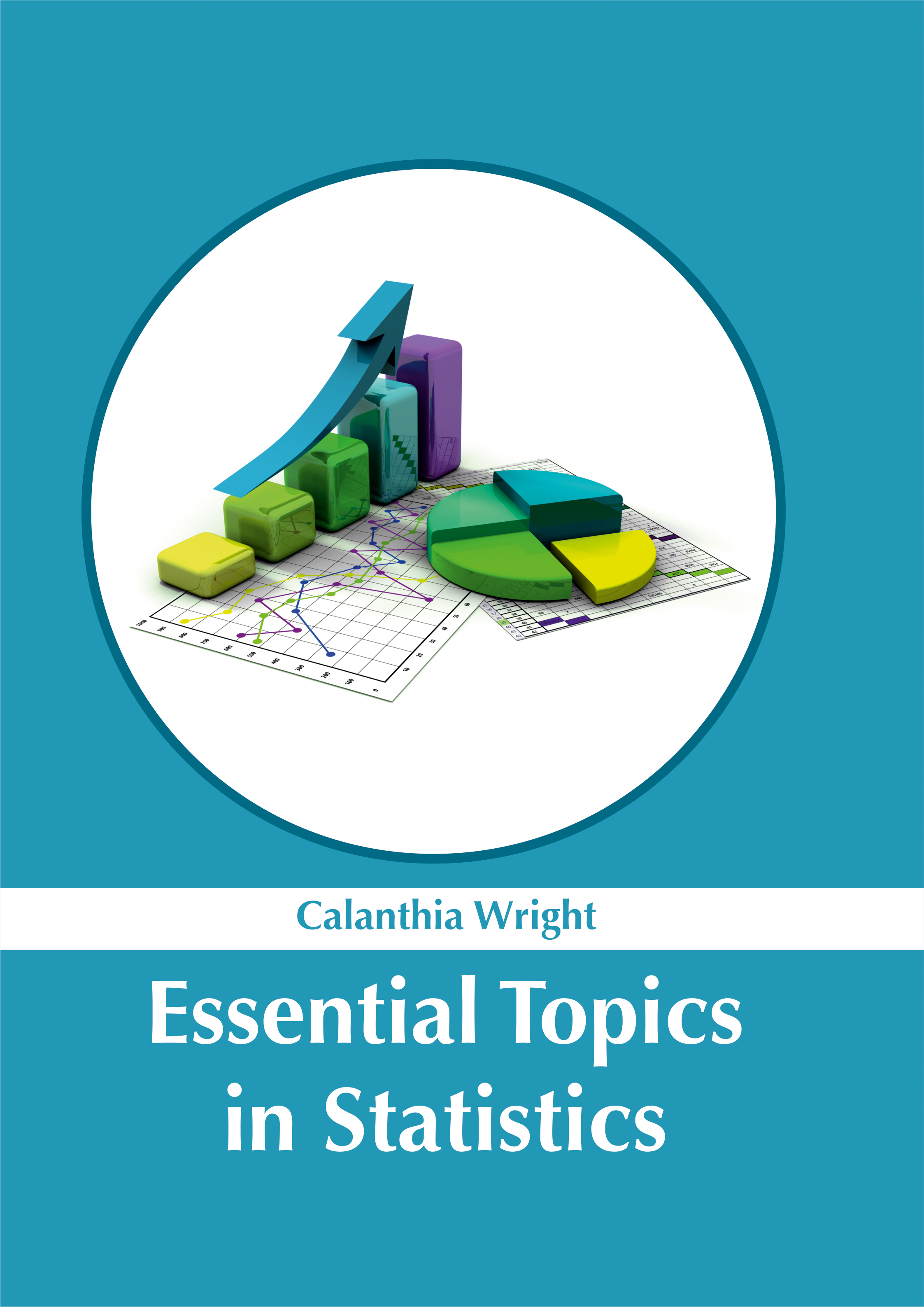Essential Topics in Statistics Author : Calanthia Wright Subject : Probability and Statistics ISBN :9781632385604 Statistics is the science of compilation of numerical data that may represent a social or physical fact. This book on statistics deals with the basic methodology that is followed to derive inferences. Read More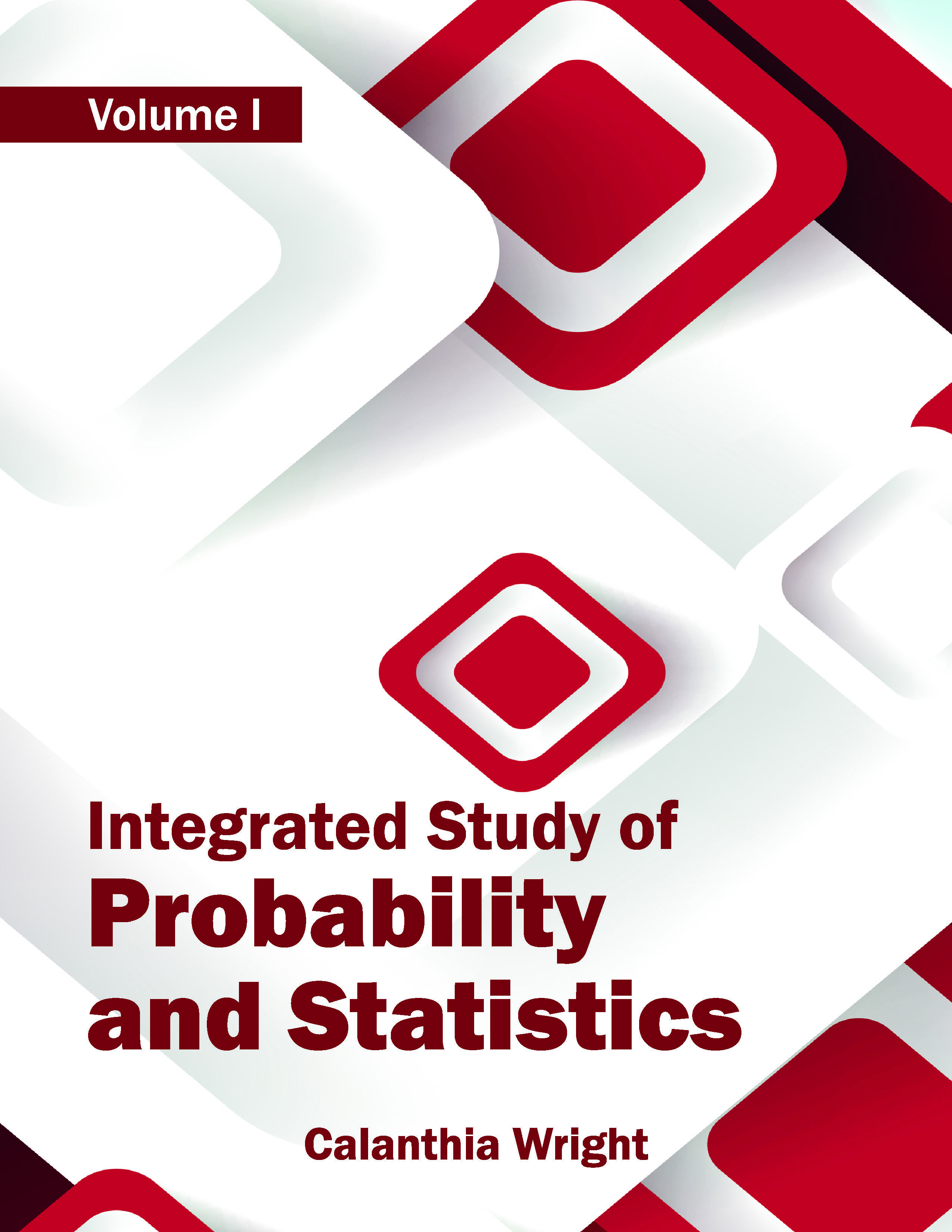Integrated Study of Probability and Statistics: Volume I Author : Calanthia Wright Subject : Probability and Statistics ISBN :9781632382962 The book attempts to help the readers understand the more advanced concepts of probability and statistics. The various theories in both disciplines are given an in-depth look. Current and proposed research Read More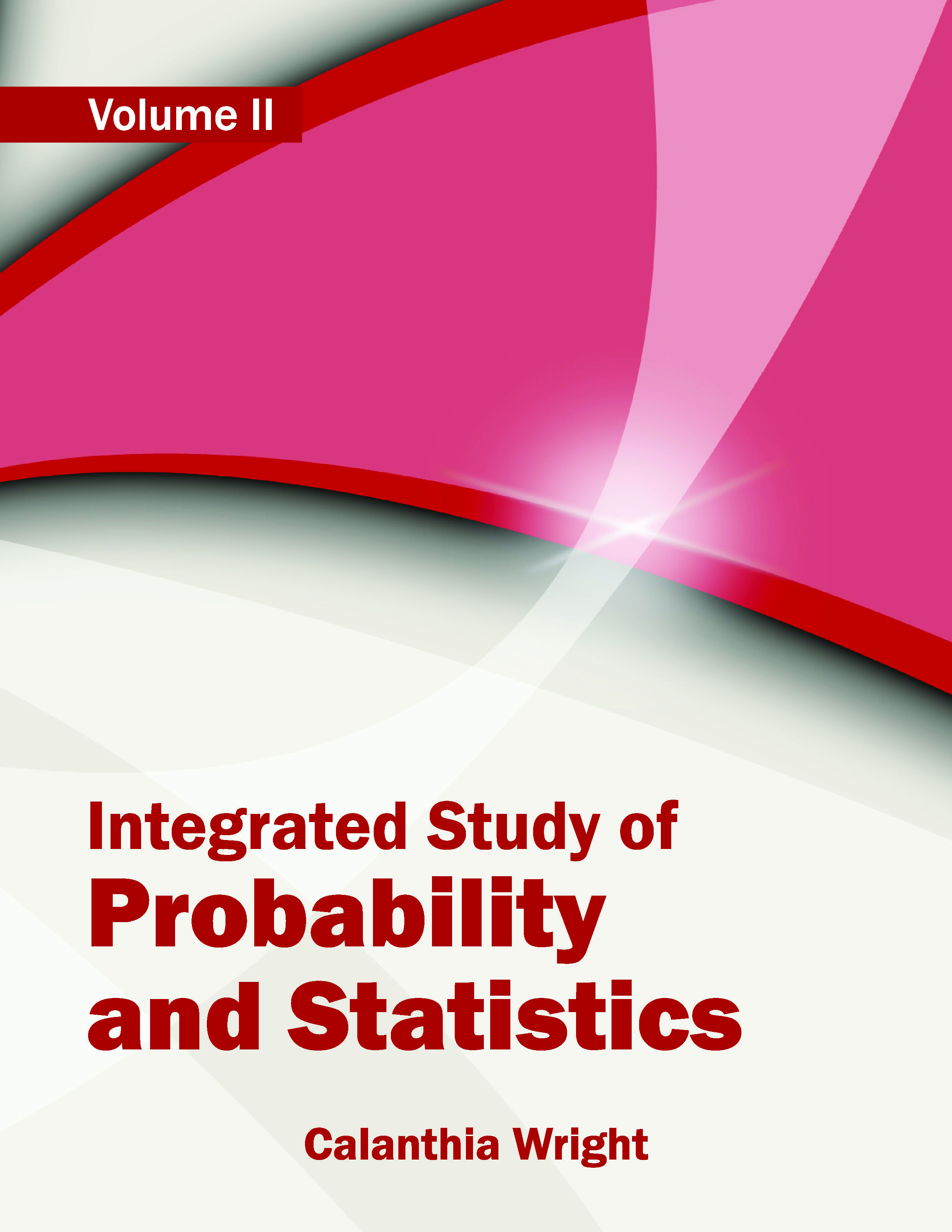Integrated Study of Probability and Statistics: Volume II Author : Calanthia Wright Subject : Probability and Statistics ISBN :9781632382979 The book attempts to help the readers understand the more advanced concepts of probability and statistics. The various theories in both disciplines are given an in-depth look. Current and proposed research Read MoreProbability and Statistics for Engineering and Sciences Author : Derek Beaven Subject : Probability and Statistics Probability and Statistics are two closely related sub-disciplines of mathematical. Statistics is a mathematical branch that deals with data collection, organization, interpretation, presentation and Read More
Results 1 - 7 of 7